# Profit and Loss Questions for Competitive Exams

Profit and Loss Questions with solution for Competitive Exams. Previous year MCQ question and Answer of SSC CGL, CHSL, CPO, IBPS Bank PO, clerk. Maths Questions are very useful for upcoming UPSSSC PET and NRA CET examinations.

### Basic Concept of C.P. and S.P. and Profit and Loss

Q.1: There is 10% loss if an article is sold at ₹ 270. Then the cost price of the article is :
a) ₹ 300
b) ₹ 270
c) ₹ 320
d) ₹ 250

Ans : a) ₹ 300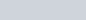$\frac {CP \times 90}{100} =270$
CP =₹ 300

Q.2: By selling an article for ₹ 450, I lose 20%. For what price should I sell it to gain 20%?
a) ₹ 490
b) ₹ 675
c) ₹ 470
d) ₹ 562.50

Ans : b) ₹ 675$\frac {CP \times 80}{100} =450$
CP = 562.50
Selling price to gain 20% =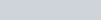$\frac {56.25 \times 120}{100} = 675$
Short Trick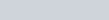$\frac {120}{80} \times 450 = 675$

Q.3: Nisha bought a number of oranges at 2 for a rupee and an equal number at 3 for a rupee. To make a profit of 20% she should sell a dozen for :
a) ₹6
b) ₹8
c) ₹10
d) ₹12

Ans: a) ₹6
Total a dozen (12) orange
CP for 6 @ 2 for one rupees = 3
CP for 6 @ 3 for one rupees = 2
CP for 12 is 3+2 = ₹ 5
For profit of 20%
SP =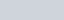$\frac {5 \times 120}{100} = 6$

Q.4: If books bought at prices from 150 to 300 are sold at prices ranging from 250 to350, what is the greatest possible profit that might be made in selling 15 books?
a) ₹ 750
b) ₹ 4250
c) ₹ 3000
d) Can not be determined

Ans : c) ₹ 3000
Minimum cost price for 15 books = 150 x 15 = 2250
Maximum SP for 15 books = 350 x 15 = 5250
Greatest possible profit = 5250 – 2250 = 3000

Q.5: By selling 33 metres of cloth, a person gains the cost of 11 metres. Find his gain%
a)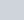$33\frac13$
b)$33\frac12$
c)$34\frac13$
d) 33%

Ans : b)$33\frac12$
Gain % =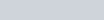$\frac {11}{33} \times 100 = 33\frac13$

Q.6: A milkman bought 70 litres of milk for 630 and added 5 litres of water. If he sells it at ₹ 9.00 per litre, his profit percentage is :
a)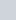$8\frac15$
b) 7
c)$8\frac25$
d)$7\frac17$

Ans : d)$7\frac17$
CP of 75 liters Milk and Water Mixture is 630
SP of 75 ltrs = 75 x 9 = 675
Profit = 675 – 630 = 45
Gain percentage =$\frac {45}{630} \times 100 = 7\frac17$

Q.7: If there is a profit of 20% on the cost price of an article, the percentage of profit calculated on its selling price will be :
a) 24
b)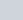$16\frac 23$
c)$8\frac13$
d) 20

Ans : b)$16\frac 23$
Tricky Solution:
CP = 100, SP = 120, Gain = 20
% of profit on SP =$\frac {20}{120} \times 100 = 16\frac23$

Q.8: If the cost price of an article is 80% of its selling price, then the profit per cent is :
a) 20%
b) 22%
c) 24%
d) 25%

Ans : d) 25%
SP = 100, CP =80, Gain = 20
Gain percent on CP =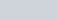$\frac {20}{80} \times 100$= 25%

Q.9: A man bought an old type writer for 1200 and spent 200 on its repair. He sold it for ₹1680. His profit per cent is :
a) 20%
b) 10%
c) 8%
d) 16%

Ans : a) 20%
total cost = 1200 +200 = 1400
Gain = 1680 -1400 = 280
Profit % =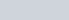$\frac {280}{1400} \times 100$ = 20%

Q.10: In terms of percentage profit, which is the best transaction?

a) I
b) II
c) III
d) IV

Ans : d) IV
Case -I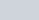$\frac {17 \times 100}{36}$ =47%
Case II – 48%, Case III – 47.5%, Case IV – 48.3%

### Gain and Loss Per cent

Q. 11: A merchant marks an article 20% above cost price. He then sells it at a discount of 20%. The sale gives him :
a) 8% loss
b) 4% profit
c) 4% loss
d) 8% profit

Ans : c) 4% loss
Let CP = 100
SP = 120
20% Discount on SP =$\frac {120 \times 20}{100}$=₹ 24
New SP = 120 – 24 = 96
Loss = 100 – 96 = 4 %

Q.12: By selling a tape-recorder for 1040 a man gains 4%. If he sells for 950, then his loss will be :
a) 5%
b) 4%
c) 4.5%
d) 9%

Ans : a) 5%
CP =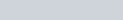$\frac{1040}{104} \times 100 =1000$
Loss = 1000 – 950 =50
% loss is$\frac{50}{1000} \times 100$ = 5%

Q.13: If the cost price of 25 chairs is equal to the selling price of 30 chairs, then the loss % is :
a) 25 %
b) 20%
c) 5%
d)$16\frac 23$

Ans : d)$16\frac 23$
Let cost of each is 1
CP of 30 chairs = ₹30, SP = ₹25
Loss 30 – 25 = 5
Loss % =$\frac {5}{30} \times 100 = 16\frac 23$%

Q.14: Ram sold two horses at the same price. In one he gets a profit of 10% and in the other he gets a loss of 10%. Then Ram gets :
a) 2% Loss
b) No Profit or Loss
c) 1% Loss
d) 1% Profit

Ans : d) 1% Loss
Here, selling prices are same, profit-loss per cent are same. In such transactions, there is always loss.
Loss % =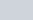$\frac {10 \times 10}{100}$= 1%

Q.15: If a shop-keeper purchases cashew nut at 250 per kg and sells it at ₹10 per 50 grams, then he will have :
a) 25% loss
b) 25% profit
c) 20% profit
d) 20% loss

Ans : d) 20% loss
CP of 1000 gm = ₹250
SP of 50 gm = ₹10
SP of 1000 gm = 50 x20 = ₹10 x20 = ₹200
Loss = 50
% loss$\frac {50 \times 100}{250}$ = 20%

Q.16: The cost price of a book is ₹150. At what price should it be sold to gain 20%?
a) ₹ 120
b) ₹ 180
c) ₹ 100
d) ₹ 80

Ans : b) ₹ 180
SP of book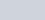$\frac {150 \times 120}{100}$ = ₹180

Q.17: A man sells 320 mangoes at the cost price of 400 mangoes. His gain per cent is :
a) 15%
b) 20%
c) 25%
d) 10%

Ans : c) 25%
Gain percent =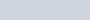$\frac {400 - 320} {320} \times 100$= 25%

### Selling and Buying : Profit and Loss Questions

Q.17: A manufacturer sells an item to a wholesale dealer at a profit of 18%. The wholesaler sells the same to a retailer at a profit of 20%. The retailer in turn sells it to a customer for ₹15,045 thereby earning a profit of 25%. The cost price of the manufacturer is :
a) ₹8000
b) ₹8500
c) ₹9000
d) ₹10000

Ans : b) ₹8500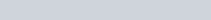$CP \times \frac {118}{100} \times \frac{120}{100} \times \frac{125}{100} = 15045$
CP = 8500

Q.18: A sold a horse to B for ₹4800 by losing 20%. B sells it to C at a price which would have given A a profit of 15%. B’s gain is :
a) ₹ 1800
b) ₹ 1900
c) ₹ 2000
d) ₹ 2100

Ans : d) ₹ 2100
CP of horse for A =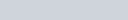$4800 \times \frac{100}{80} = 6000$
SP for B =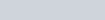$\frac {6000 \times 115}{100} = 6900$
Profit for B is 6900 – 4800 = 2100

Q.19: A man sold 20 apples for 100 and gained 20%. How many apples did he buy for ₹100?
a) 20
b) 22
c) 24
d) 25

Ans : c) 24
Assume CP of 20 apples is A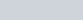$\frac {A \times 120}{100} =100$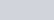$A = \frac {250}{3}$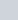$\frac {250}{3}$ = 20 apples$100 = \frac {20 \times 3 \times 100}{250}$ = 24 apples

Q.20: If I purchased 11 books for ₹100 and sold 10 books for ₹110, the percentage of profit per book sold is :
a) 10%
b) 11.5%
c) 17.3%
d) 21%

Ans : d) 21%
If a articles are bought for x and b articles are sold for y, then
Gain per cent =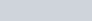$( \frac{ya - xb}{xb}) \times 100$
=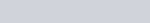$\frac {(11\times 110) - (10\times 100)}{10\times100} \times 100$
=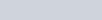$\frac {1210 -1000}{1000} \times 100$ = 21%

### Profit and Loss Questions based on Tricks for Competitive Exams

Q.21: A shopkeeper gains 20% while buying the goods and 30% while selling them. Find his total gain per cent :
a) 50%
b) 36%
c) 56%
d) 40%

Ans : c) 56%
For two consecutive gains of x % and y %,
Effective gain =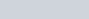$(x+y+\frac{xy}{100})$%
Total Gain =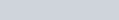$(20+30+\frac{20 \times30}{100})$ =56%

Q.22: A grocery dealer cheats to the extent of 10% while buying as well as selling by using false weight. What is his increase in the profit %?
a) 20%
b) 21%
c) 22%
d) 24%

Ans : b) 21%
Gain % =$(10+10+\frac {10+10}{100})$% = 21%

Q.23: The salary of a person is increased by 20%, then it is decreased by 20%. The change in his salary is :
a) 4% decreased
b) 4% increased
c) 8% decreased
d) No change

Ans : a) 4% decreased
If the value of a number is first increased by x % and later decreased
by x%, the net change is always a decrease which is equal to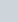$\frac {x^2}{100}$%
Decrease =$\frac {20 \times 20}{100}$ = 4%

### Based on Selling an Article and Interchanging its Values

Q.24: By selling 90 ball pens for ₹160 a person loses 20%. The number of ball pens, which should be sold for ₹ 96 so as to have a profit of 20% is :
a) 36
b) 37
c) 46
d) 47

Ans : a) 36
CP of 90 pens =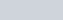$\frac {100}{80}\times 160$ = ₹200
SP of 90 pens for gain of 20% =$\frac{200\times120}{100}$ = ₹240
₹ 240 = 90 pens
₹ 96 =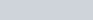$\frac {90}{240}\times 96 = 36$

Q.25: By selling a table for ₹1140, a man loses 5% in order to gain 5%, the table must be sold for :
a) ₹1260
b) ₹1320
c) ₹ 1180
d) ₹1250

Ans : a) ₹1260
CP of table =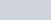$\frac {1140 \times 100}{95}$ =₹1200
SP for a gain of 5% =$\frac {1200\times 105}{100}$= ₹1260

### Marked Price : Profit and Loss Questions for Competitive Exams

Q.26: Mohan purchased a bag with 20 per cent discount on the labelled price. He sold it at 40 per cent profit on the price he bought. The percentage of profit on the labelled price is :
a) 20%
b) 12%
c) 18%
d) 24%

Ans : b) 12%
Let Labelled Price =100
Mohan CP = 80
Mohan SP =$\frac{80\times140}{100}$= 112
% profit on labelled price 112 -100 = 12%

Q.27: A tradesman allows a discount of 15% on the marked price. How much above the cost price must he mark his goods as to gain 19%?
a) 34%
b) 40%
c) 25%
d) 30%

Ans : b) 40%
Let CP =100
SP for 19% gain = 119
Let Marked Price is A, then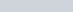${A \times 85}{100}$ = 119
=> A = 140
Marked price =(140 – 100)% = 40% of above cost price

### Loss/Gain Per cent is equal to the CP/SP

Q.28: Profit after selling a commodity for ₹524 is the same as loss after selling it for ₹452. The cost price of the commodity is :
a) ₹ 480
b) ₹ 500
c) ₹ 488
d) ₹ 485

Ans : c) ₹ 488
Let CP = ₹x
525 – x = x-452
=> 2x = 976
=> x= 488

### Profit and Loss, when Articles are sold in a Circular Chain

Q.29: A sells an article to B at a gain of 10%. B sells it to C at a gain of 5%. If C pays ₹462 for it what did it cost to A?
a) ₹500
b) ₹450
c) ₹400
d) ₹600

Ans : c) ₹400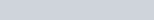$CP \times \frac{110}{100}\times \frac{105}{100} =462$
=>CP =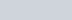$\frac {462\times100\times100}{110\times105}$= ₹400

Q.30: The price of a jewel, passing through three hands, rises on the whole by 65%. If the first and the second sellers earned 20% and 25% profit respectively, the profit earned by the third seller is :
a) 20%
b) 15%
c) 10%
d) 5%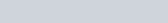$\frac{120}{100}\times\frac{125}{100}\times \frac{(100+x)}{100} = \frac{165}{100}$
=>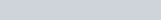$(100+x) = \frac{165\times100\times100}{120\times125}$
=>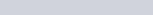$100 + x = 110, x= 10$%